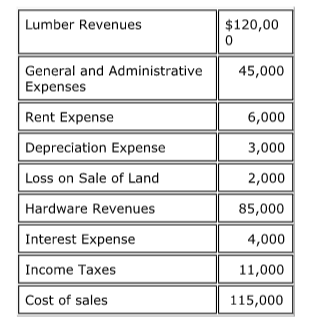# Problem: Consider the following information provided in no particular order: What is the dollar amount of the operating income? a. $36,000 b.$15,000 c. $17,000 d.$205,000

###### Problem Details

Consider the following information provided in no particular order:

What is the dollar amount of the operating income?

a. $36,000 b.$15,000

c. $17,000 d.$205,000Frequently Asked Questions

What scientific concept do you need to know in order to solve this problem?

Our tutors have indicated that to solve this problem you will need to apply the Multi-step Income Statement concept. You can view video lessons to learn Multi-step Income Statement. Or if you need more Multi-step Income Statement practice, you can also practice Multi-step Income Statement practice problems.

What is the difficulty of this problem?

Our tutors rated the difficulty ofConsider the following information provided in no particular...as medium difficulty.